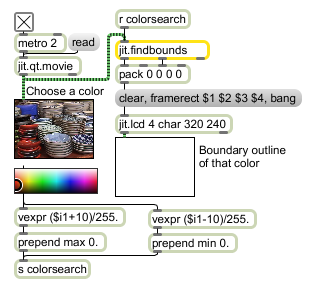jit.findbounds

Calculate bounding dimensions for a range of values

Description

The jit.findbounds object scans a matrix for values in the range [ min, max ] and sends out the minimum and maximum points that contain values in the range [ min, max ]. The minimum point is sent as a list out the leftmost outlet, and the maximum point is sent as a list out the second outlet. If both points are all -1 values, then there are no points in the range [ min, max ] in the matrix.

Matrix Operator

matrix inputs:1, matrix outputs:0

Attributes

Name Type g/s Description
boundmax int (get) The maximum bounding point
boundmin int (get) The minimum bounding point
max float The maximum value to search for (default = all 0.)
min float The minimum value to search for (default = all 0.)

Examples